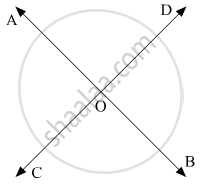# Two Straight Line Ab and Cd Intersect One Another at the Point O. If ∠Aoc + ∠Cob + ∠Bod = 274°, Then ∠Aod = - Mathematics

MCQ

Two straight line AB and CD intersect one another at the point O. If ∠AOC + ∠COB + ∠BOD = 274°, then ∠AOD =

•  86°

•  90°

•  94°

• 137°

#### Solution

Let us draw the following diagram showing two lines AB and CD intersecting at a point O.Thus,

$\angle \text { AOD}, \angle \text { AOC }, \angle \text { COB } \text { and } \angle \text { BOD }$ form a complete angle, that is the sum of these four angle is 360°.

That is,

$\angle \text { AOD }, \angle \text { AOC }, \angle \text { COB } \text { and } \angle \text { BOD }$   = 360°   .......(1)

It is given that

∠AOC + ∠COB  +BOD = 274°                        ...(ii)

Subtracting (ii) from (i), we get:

∠AOD =360° - 274°

∠AOD = 86°

Concept: Concept of Parallel Lines
Is there an error in this question or solution?

#### APPEARS IN

RD Sharma Mathematics for Class 9
Chapter 10 Lines and Angles
Q 2 | Page 51